Homework Help Question & Answers

# 12. Market equilibrium and disequilibrium The following graph shows the monthly demand and supply curves in...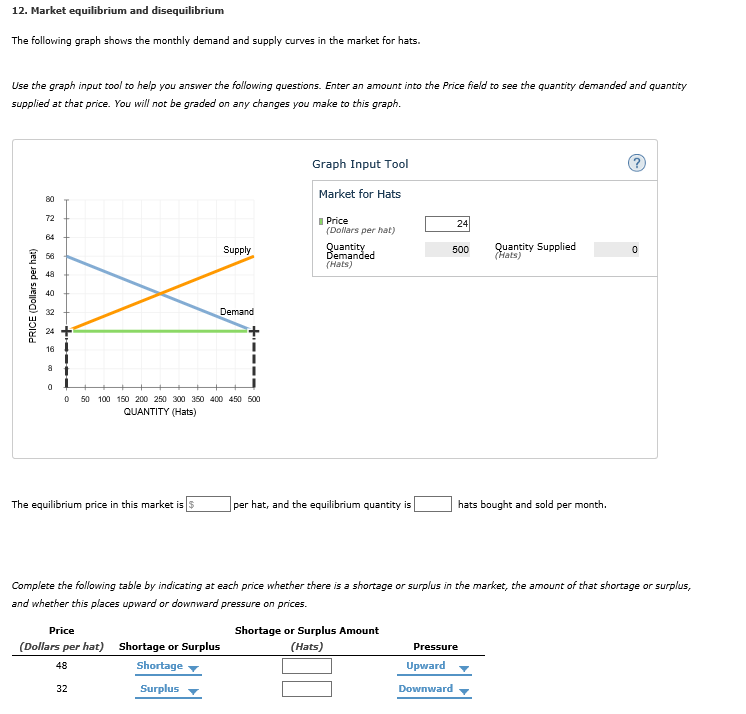12. Market equilibrium and disequilibrium

The following graph shows the monthly demand and supply curves in the market for hats.

Use the graph input tool to help you answer the following questions. Enter an amount into the Price field to see the quantity demanded and quantity supplied at that price. You will not be graded on any changes you make to this graph.

The equilibrium price in this market is 5 per hat, and the equilibrium quantity is hats bought and sold per month.

Complete the following table by indicating at each price whether there is a shortage or surplus in the market, the amount of that shortage or surplus, and whether this places upward or downward pressure on prices.

#### Homework Answers

Answer #2 ✔ Recommended Answer

Shortage is where demand is greater than supply when this happens the pressure is upward on price because demand is higher than supply due to shortage the market will not be able to supply much and thus will charge higher

Now in case of surplus when supply is greater than demand the pressure in downward on price because the market is already filled with such alarge amount kf products that arent even required thus inventories will be pilled up and thus price will fall down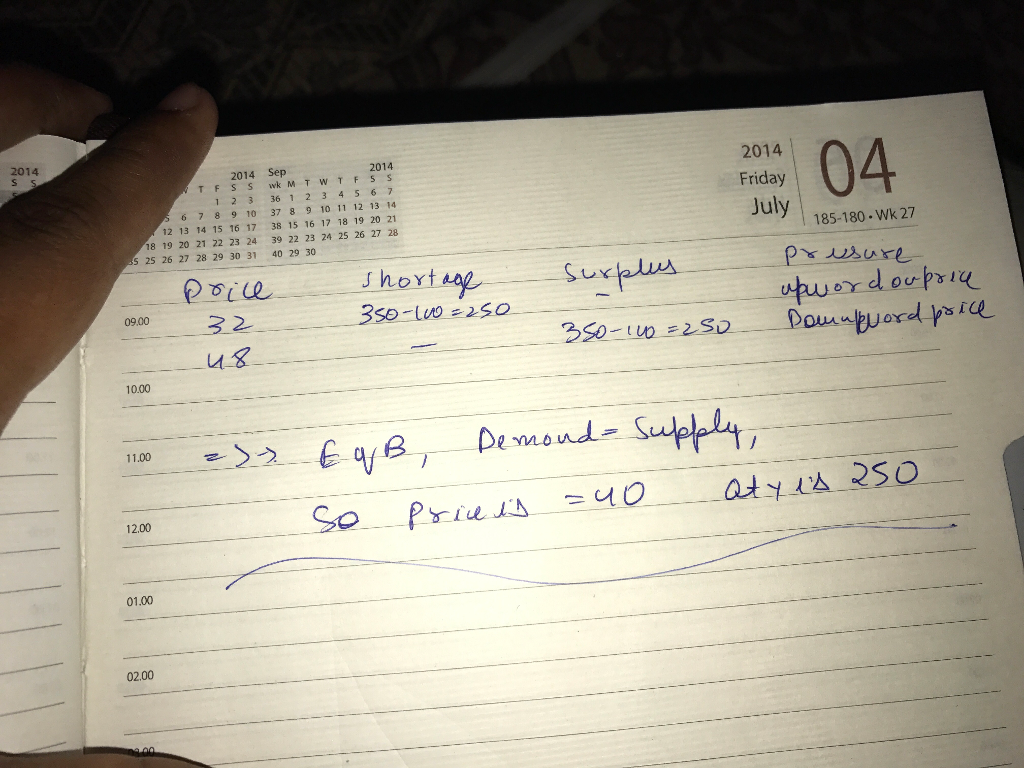Add a comment
Answer #1

The equilibrium in the market is calculated from the intersection of the demand curve and the supply curve. As evident from the graph, the intersection point is (250,40).

Thus equilibrium price in the market is \$40 per hat and equilibrium quantity is 250 hats bought and sold per month.

At P = 48, Supply is more than demand (can be seen from the graph) (Surplus)

Demand = 100 and supply = 400

Surplus amount = 400 -100 = 300

Pressure on price is downward.

At P = 32, there is an excess demand(Shortage)

Demand = 400 and supply =100

Shortage = excess demand = 400 -100 = 300

Pressure on price is upward.

Know the answer?
Your Answer:

#### Post as a guest

Your Name:

What's your source?

#### Earn Coin

Coins can be redeemed for fabulous gifts.

Not the answer you're looking for? Ask your own homework help question. Our experts will answer your question WITHIN MINUTES for Free.
Similar Homework Help Questions
• ### Please solve above questions. 12. Market equilibrium and disequilibrium The following graph shows the monthly demand...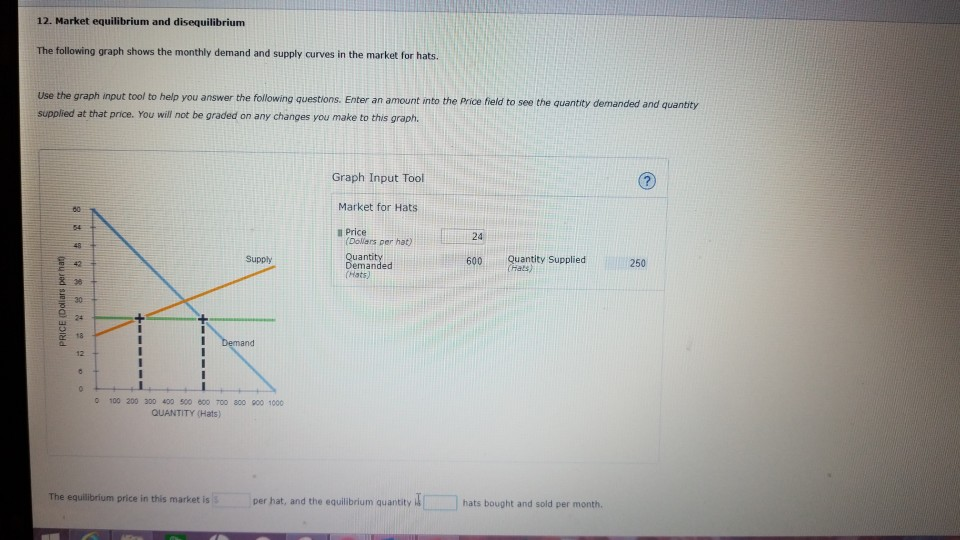Please solve above questions. 12. Market equilibrium and disequilibrium The following graph shows the monthly demand and supply curves in the market for hats. Use the graph input tool to help you answer the following questions. Enter an amount into the Price f supplied at that price. You will not be graded on any changes you make to this graph. field to see the quantity demanded and quantity Graph Input Tool Market for Hats l Price 24 (Dolars per hat)...

• ### 12: Market equilibrium and disequilibrium Homework (Ch 04) 12. Market equilibrium and disequilibrium The following graph...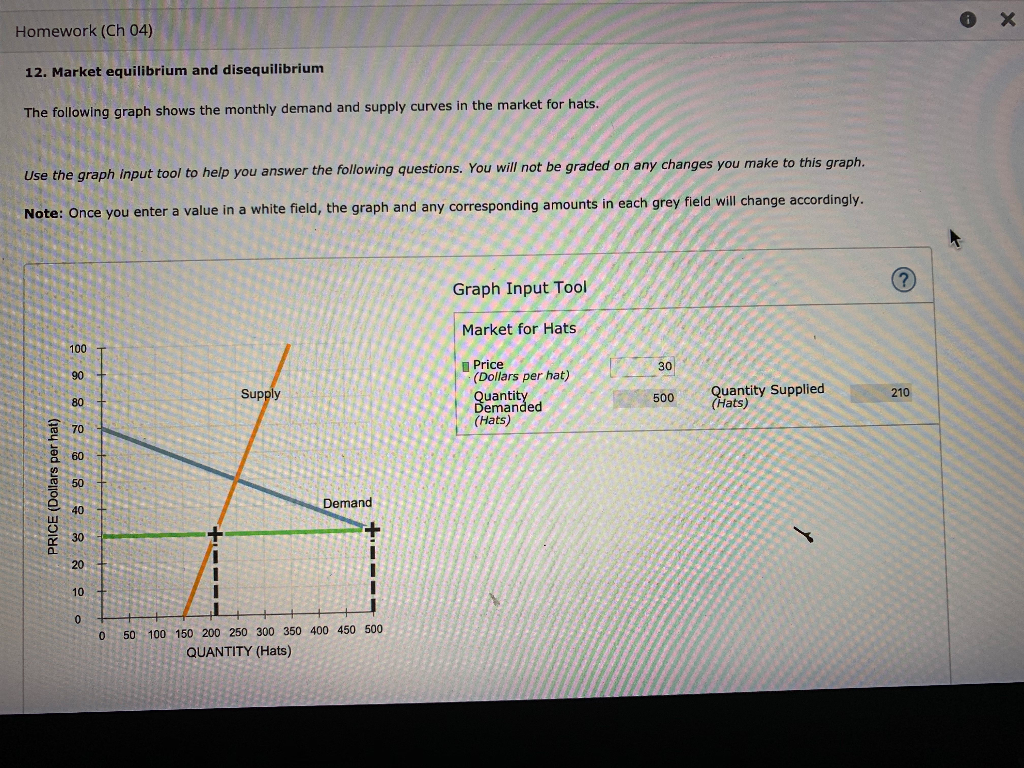12: Market equilibrium and disequilibrium Homework (Ch 04) 12. Market equilibrium and disequilibrium The following graph shows the monthly demand and supply curves in the market for hats. Use the graph input tool to help you answer the following questions. You will not be graded on any changes you make to this graph. Note: Once you enter a value in a white field, the graph and any corresponding amounts in each grey field will change accordingly. . Graph Input Tool...

• ### 12. Market equilibrium and disequilibrium The following graph shows the monthly demand and supply curves in...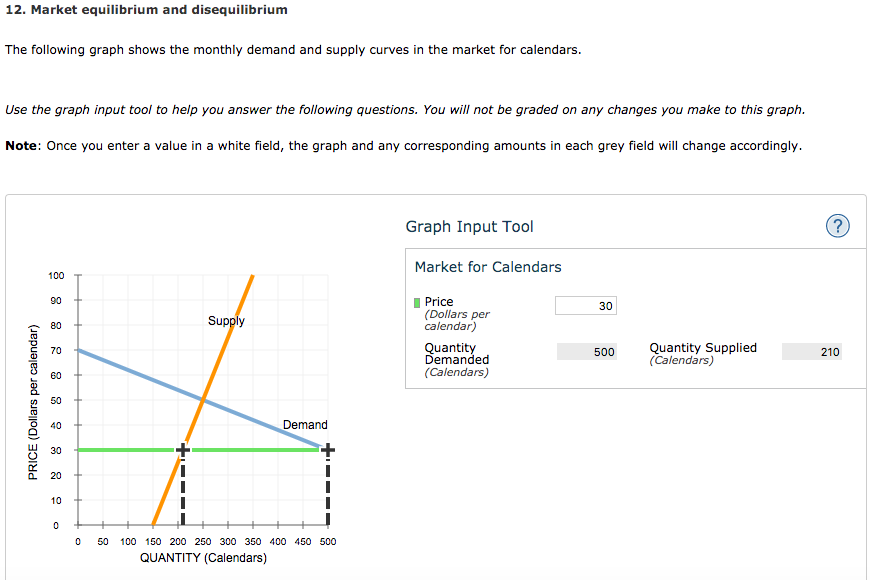12. Market equilibrium and disequilibrium The following graph shows the monthly demand and supply curves in the market for calendars. Use the graph input tool to help you answer the following questions. You will not be graded on any changes you make to this graph. Note: Once you enter a value in a white field, the graph and any corresponding amounts in each grey field will change accordingly. Graph Input Tool Market for Calendars 100 90 Price 30 (Dollars per...

• ### 12. Market equilibrium and disequilibrium The following graph shows the monthly demand and supply curves in...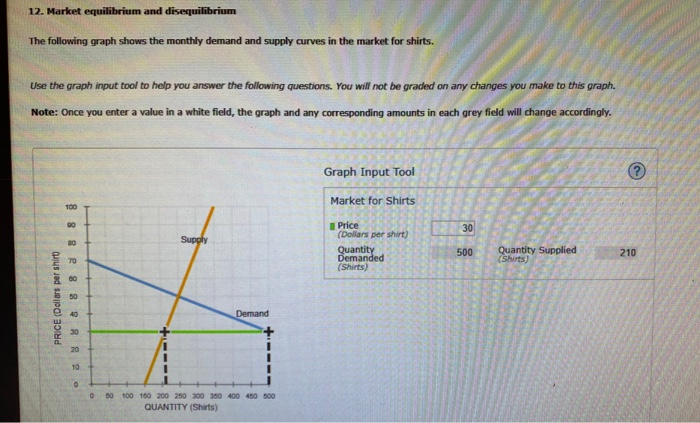12. Market equilibrium and disequilibrium The following graph shows the monthly demand and supply curves in the market for shirts. Use the graph input tool to help you answer the following questions. You will not be graded on any changes you make to this graph. Note: Once you enter a value in a white field, the graph and any corresponding amounts in each grey field will change accordingly. Graph Input Tool Market for Shirts 8 8 30 8 Price (Dollars per shirt Quantity Demanded...

• ### ack to Assignment Keep the Highest: /2 ttempts: 12. Market equilibrium and disequilibrium The following graph shows the monthly demand and supply curves in the market for keyboards. Use the gr...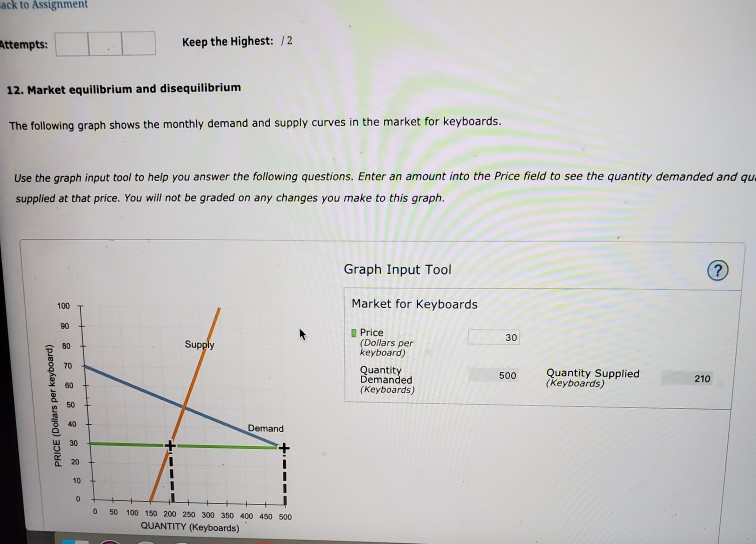ack to Assignment Keep the Highest: /2 ttempts: 12. Market equilibrium and disequilibrium The following graph shows the monthly demand and supply curves in the market for keyboards. Use the graph input tool to help you answer the following questions. Enter an amount into the Price field to see the quantity demanded and qu supplied at that price. You will not be graded on any changes you make to this graph Graph Input Tool Market for Keyboards 100 90 Price...

• ### The following graph shows the monthly demand and supply curves in the market for calendars. Use...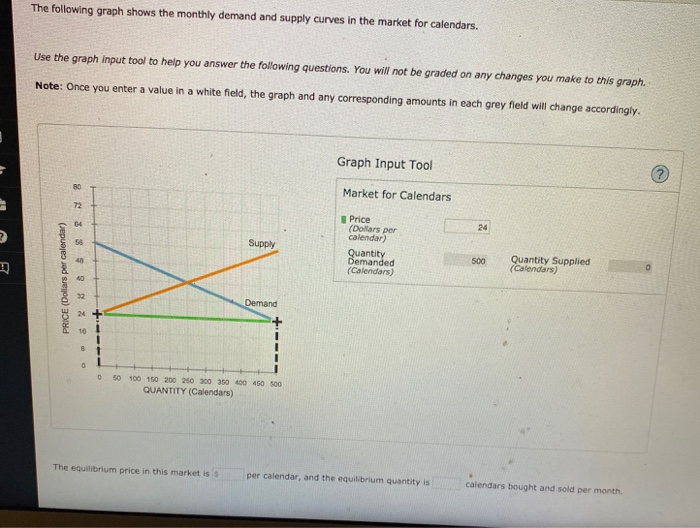The following graph shows the monthly demand and supply curves in the market for calendars. Use the graph input tool to help you answer the following questions. You will not be graded on any changes you make to this graph. Note: Once you enter a value in a white field, the graph and any corresponding amounts in each grey field will change accordingly. Graph Input Tool Market for Calendars 8 Supply Price (Dollars per calendar) Quantity Demanded (Calendars 500 Quantity...

• ### ack to Assignment Keep the Highest: /2 ttempts: 12. Market equilibrium and disequilibrium The following graph...ack to Assignment Keep the Highest: /2 ttempts: 12. Market equilibrium and disequilibrium The following graph shows the monthly demand and supply curves in the market for keyboards. Use the graph input tool to help you answer the following questions. Enter an amount into the Price field to see the quantity demanded and qu supplied at that price. You will not be graded on any changes you make to this graph Graph Input Tool Market for Keyboards 100 90 Price...

• ### 12. Market equilibrium and disequilibrium Use the graph input tool to help you answer the following...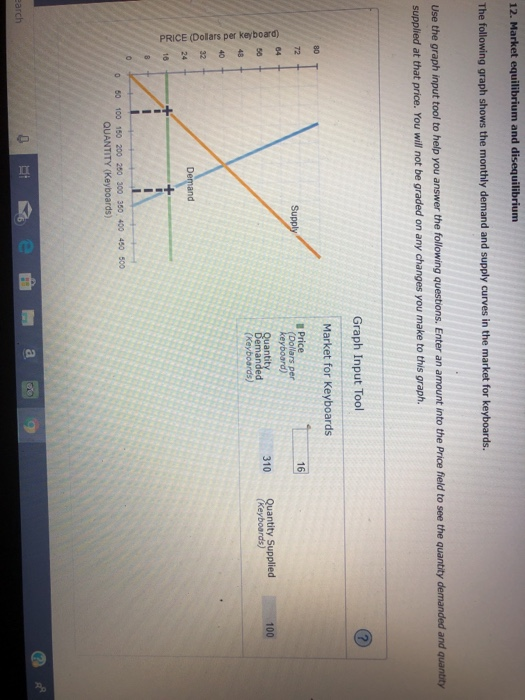12. Market equilibrium and disequilibrium Use the graph input tool to help you answer the following questions. Enter an amount into the Price field to see the quantity supplied at that price. You will not be graded on any changes you make to this graph. and quantity Graph Input Tool Market for Keyboards antit emanded 310 Quantity Supplied 100 2 48 O 32 U 24 o 50 100 150 200 250 300 350, 400 40 500 QUANTITY (Keyboards) u 24...

• ### 12. Market equilibrium and disequilibrium The following graph shows the monthly demand and supply curves in...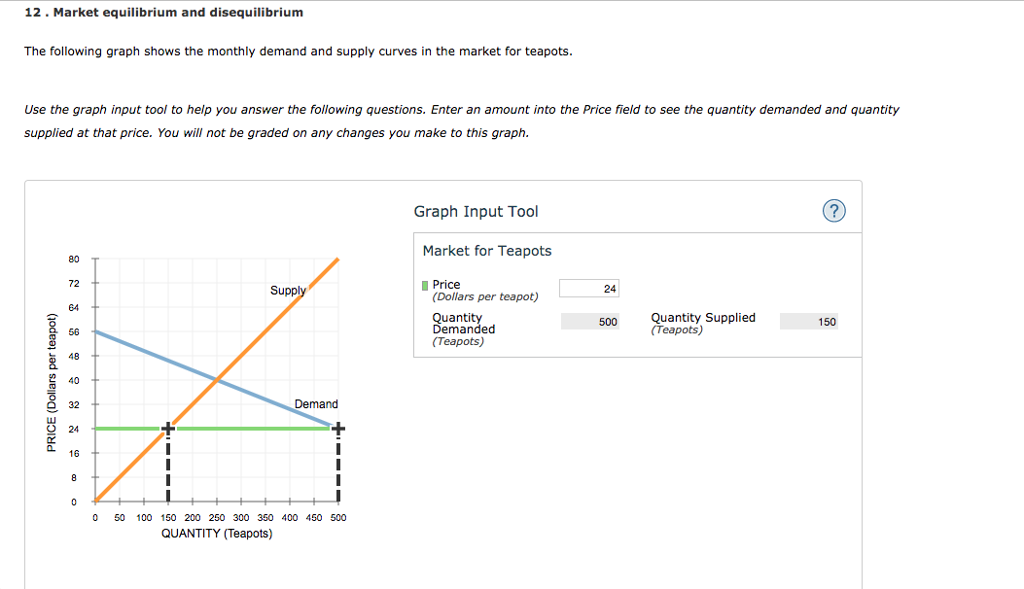12. Market equilibrium and disequilibrium The following graph shows the monthly demand and supply curves in the market for teapots Use the graph input tool to help you answer the following questions. Enter an amount into the Price field to see the quantity demanded and quantity supplied at that price. You will not be graded on any changes you make to this graph Graph Input Tool Market for Teapots 72 Price (Dollars per teapot) Su 24 64 Quantit Demanded (Teapots)...

• ### 3. Market Equilibrium and Disequilibrium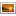The following graph shows the monthly demand and supply curves in the market for keyboards. Use the graph input tool to help you answer the following questions. You will not be graded on any changes you make to this graph. Note: Once you enter a value in a white field, the graph and any corresponding amounts in each grey field will change accordingly. The equilibrium price in this market is  per keyboard, and the equilibrium quantity is   keyboards bought and sold...